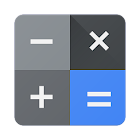• 검색 유형

모든 Android 애플리케이션 카테고리

모든 Android 게임 카테고리# Calculator

by: 61K 8.6

8.6 사용자
평가 등급

## 스크린샷

설명

Calculator provides simple and advanced mathematical functions in a beautifully designed app.

• Perform basic calculations such as addition, subtraction, multiplication, and division
• Do scientific operations such as trigonometric, logarithmic, and exponential functions

: 60.981 리뷰

"우수"

8.6